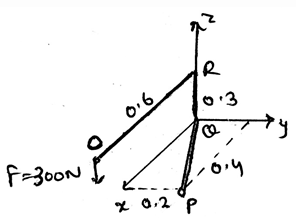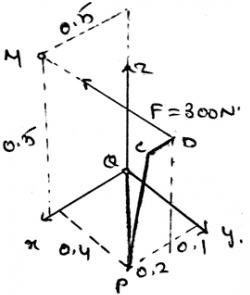# Test: Bending Moment Diagram

## 15 Questions MCQ Test Engineering Mechanics | Test: Bending Moment Diagram

Description
This mock test of Test: Bending Moment Diagram for Mechanical Engineering helps you for every Mechanical Engineering entrance exam. This contains 15 Multiple Choice Questions for Mechanical Engineering Test: Bending Moment Diagram (mcq) to study with solutions a complete question bank. The solved questions answers in this Test: Bending Moment Diagram quiz give you a good mix of easy questions and tough questions. Mechanical Engineering students definitely take this Test: Bending Moment Diagram exercise for a better result in the exam. You can find other Test: Bending Moment Diagram extra questions, long questions & short questions for Mechanical Engineering on EduRev as well by searching above.
QUESTION: 1

### Which of the following is correct in bending moment diagram w.r.t the moment (M) of the force (F) acting on the body at a distance L from the axis of the rotation?

Solution:

The moment of the force about the axis of rotation by the application of the force on the body is given by the cross product of both. If the force not perpendicular to the axis, and making angle θ then cosine form of angle is used. As usually used in the cross product.

QUESTION: 2

### What does the moment of the force measure in the bending moment diagram?

Solution:

The moment of the force measures the tendency of the rotation of the body along any axis, whether it be the centroid axis of the body, or any of the outside axis. The couple moment is produced by two forces, not by a single force. The total work done is the dot product of force and distance not the cross.

QUESTION: 3

### Determine the moment’s magnitude produce by the force as shown in the diagram, which tends to rotate the rod ORQP along QP.Solution:

The use of the formula A.(rxF) gives the answer. In which A is 0.89i + 0.447j m. And the force is 300N, which is being applied at the end of the rod. Thus, after finding the equation of the axis and then replacing it in the equation shown above we get the answer. Actually, the main task is to know the axis equation in the vector form. Then get the magnitude of the moment.

QUESTION: 4

The moment of the force in bending moment diagram is the product of the force and the perpendicular distance of the axis and the point of action of the force.

Solution:

The moment is the product of the force applied to the body and the perpendicular distance of the point of action of the force to the axis about which the body is being rotated. That is the moment is the cross product of the force and the distance between the axis and the point of action.

QUESTION: 5

If any force is applied in the direction of the positive x-axis, and there are three different point in bending moment diagram on which the moment of this force is to be calculated. Then if these three points are on the positive side of the y-axis with varying distance, then what will be the direction of the moment caused by the force to the individual point?

Solution:

If you will apply he right hand rule on the system given then the right answer is the positive z-axis. Which is because the force is lying on the x-axis and is heading towards the positive infinity of the x-axis. And the points are in the positive y-axis. Apply the rule, and get the direction.

QUESTION: 6

Determine the moment of the force F along the segment QP of the pipe assembly shown in the figure.Solution:

The use of the formula A.(rxF) gives the answer. In which A is 0.6i + 0.8j m and the r is 0.5i + 0.5k. And the force is 300N, which is being applied at the end of the rod. Thus, after finding the equation of the axis and then replacing it in the equation shown above we get the answer. Actually, the main task is to know the axis equation in the vector form. Then get the magnitude of the moment.

QUESTION: 7

Which of the following is true for bending moment diagram?

Solution:

The moment is the vector quantity. Thus the value of the total moment caused by various forces acting on the body is the vector sum of all the vectors. Also the moments are not perpendicular to each other, unless it is specified. Thus assumptions cant be taken for the direction of the moment.

QUESTION: 8

If you are getting to know about the direction of the moment caused by the force applied on the body by using your wrist and curling it in the direction of the rotation then which of the following is not right for bending moment diagram?

Solution:

The curled hand represents various thing. The direction of the moment axis is given by the thumb. The direction of the force is given by the fingers. As we place the fingers on the force and curl towards the rotational direction of the body about the axis.

QUESTION: 9

If the rotation is clockwise in this page, suppose, then in which direction will the thumb project if you curl your hand in the same direction of the rotation for bending moment diagram?

Solution:

As the curling will give the direction perpendicular to the paper. But it does depend upon the rotation sense. In this example, the sense is clockwise. Thus the thumb goes into the paper. That is it goes away from the viewer. Thus the answer.

QUESTION: 10

The tendency of rotation of the body along any axis in bending moment diagram is also called ___________

Solution:

The tendency of rotation of the body along any axis also called the torque. It is the moment of the force acting perpendicular to the direction of the axis of rotation. If the axis and the force are meeting at any point then there is no moment applied by the force.

QUESTION: 11

What does FLsinθ means/represents in bending moment diagram for the moment (M) of the force (F) acting on the body at a distance L from the axis of the rotation?

Solution:

The moment of the force about the axis of rotation by the application of the force on the body is given by the cross product of both. If the force not perpendicular to the axis, and making angle θ then cosine form of angle is used. Thus, FLsinθ represents the magnitude of the moment.

QUESTION: 12

The moment axis, force and the perpendicular distance in the bending moment diagram is lying in____________.

Solution:

The moment axis, force and the perpendicular distance is lying in the three dimensional Cartesian. It doesn’t lye on the single plane. It also doesn’t lye in a single line. Nor in the direction of the force. Thus they all lye in the planes which are perpendicular to each other.

QUESTION: 13

If a force applied at any point in its line of action and is still creating the same moment about any fixed point say P, then the force is said to be sliding vector. What is the name of this property in bending moment diagram?

Solution:

If a force applied at any point in its line of action and is still creating the same moment about any fixed point say P, then the force is said to be sliding vector. This is because the moment of the force which is acting on its line of axis at the point P is same throughout. This is known as the principle transmissibility of the force.

QUESTION: 14

The basic way of getting the direction of the moment caused by the force in bending moment diagram is:

Solution:

The basic way of doing so is to use right hand rule and not the left hand rule. The direction of the moment axis is given by the thumb. The direction of the force is given by the fingers. As we place the fingers on the force and curl towards the rotational direction of the body about the axis.

QUESTION: 15

If a force applied at any point in its line of action and is still creating the same moment about any fixed point say P, then the force in bending moment diagram is said to be______________

Solution:

If a force applied at any point in its line of action and is still creating the same moment about any fixed point say P, then the force is said to be sliding vector. This is because the moment of the force which is acting on its line of axis at the point P is same throughout. Whatever be the direction of the distance.